Next: Critical Frequencies and Gravitational Previous: Extragalactic Gravitational Wave Background

## Gravitational Wave Background from Individual Galaxies

The number of binaries per frequency decade per solid angle from a separate stellar system (galaxy or cluster of galaxies) iswhere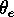is the angular size of the object,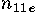is the total number of stars entering this object, which is assumed to be homogeneous in surface density. For example, for the Andromeda galaxy M31 (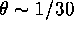rad,) one getstherefrom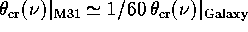(see equation (15.5.1)). The detector's beam halfwidth as a function of frequency is shown in FigureĀ41. If the angular size of the galaxy is less than the angular resolution of the detector, the critical frequency is independent of the angular resolution,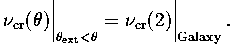Mike E. Prokhorov
Sat Feb 22 18:38:13 MSK 1997